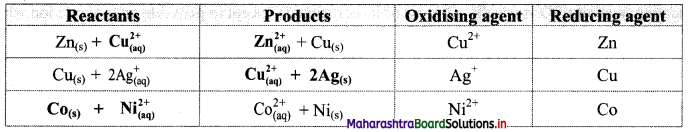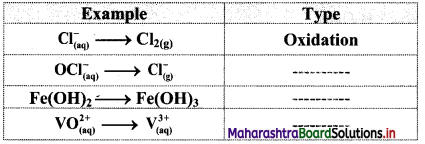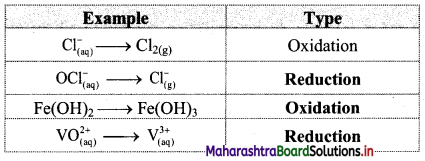# Maharashtra Board Class 11 Chemistry Solutions Chapter 6 Redox Reactions

Balbharti Maharashtra State Board 11th Chemistry Textbook Solutions Chapter 6 Redox Reactions Textbook Exercise Questions and Answers.

## Maharashtra State Board 11th Chemistry Solutions Chapter 6 Redox Reactions

1. Choose the most correct option

Question A.
Oxidction numbers of Cl atoms marked as Cla and Clb in CaOCl2 (bleaching powder) are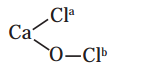a. zero in each
b. -1 in Cla and +1 in Clb
c. +1 in Cla and -1 in Clb
d. 1 in each
b. -1 in Cla and +1 in Clb

Question B.
Which of the following is not an example of redox reacton ?
a. CuO + H2 → Cu + H2O
b. Fe2O3 + 3CO2 → 2Fe + 3CO2
c. 2K + F2 → 2KF
d. BaCl2 + H2SO4 → BaSO4 + 2HCl
d. BaCl2 + H2SO4 → BaSO4 + 2HCl

Question C.
A compound contains atoms of three elements A, B and C. If the oxidation state of A is +2, B is +5 and that of C is -2, the compound is possibly represented by
a. A2(BC3)2
b. A3(BC4)2
c. A3(B4C)2
d. ABC2
b. A3(BC4)2

Question D.
The coefficients p, q, r, s in the reaction
$$\mathrm{pCr}_{2} \mathrm{O}_{7}^{2-}$$ + q Fe2⊕ → r Cr3⊕ + s Fe3⊕ + H2O respectively are :
a. 1, 2, 6, 6
b. 6, 1, 2, 4
c. 1, 6, 2, 6
d. 1, 2, 4, 6
c. 1, 6, 2, 6Question E.
For the following redox reactions, find the correct statement.
Sn2⊕ + 2Fe3⊕ → Sn4⊕ + 2Fe2⊕
a. Sn2⊕ is undergoing oxidation
b. Fe3⊕ is undergoing oxidation
c. It is not a redox reaction
d. Both Sn2⊕ and Fe3⊕ are oxidised
a. Sn2⊕ is undergoing oxidation

Question F.
Oxidation number of carbon in H2CO3 is
a. +1
b. +2
c. +3
d. +4
d. +4

Question G.
Which is the correct stock notation for magenese dioxide ?
a. Mn(I)O2
b. Mn(II)O2
c. Mn(III)O2
d. Mn(IV)O2
d. Mn(IV)O2

Question I.
Oxidation number of oxygen in superoxide is
a. -2
b. -1
c. –$$\frac {1}{2}$$
d. 0
c. –$$\frac {1}{2}$$Question J.
Which of the following halogens does always show oxidation state -1 ?
a. F
b. Cl
c. Br
d. I
a. F

Question K.
The process SO2 → S2Cl2 is
a. Reduction
b. Oxidation
c. Neither oxidation nor reduction
d. Oxidation and reduction.
a. Reduction

2. Write the formula for the following compounds :
A. Mercury(II) chloride
B. Thallium(I) sulphate
C. Tin(IV) oxide
D. Chromium(III) oxide
i. HgCl2
ii. Tl2SO4
iii. SnO2
iv. Cr2O3Question A.
In which chemical reaction does carbon exibit variation of oxidation state from -4 to +4 ? Write balanced chemical reaction.
In combustion of methane, carbon exhibits variation from -4 to +4. The reaction is as follows:
CH4 + 2O2 → CO2 + 2H2O
In CH4, the oxidation state of carbon is -4 while in CO2, the oxidation state of carbon is +4.

Question B.
In which reaction does nitrogen exhibit variation of oxidation state from -3 to +5 ?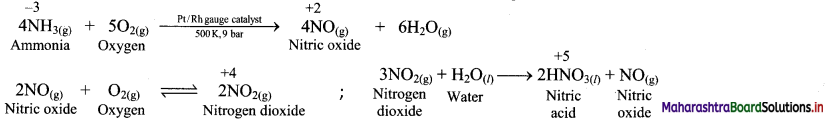C. Calculate the oxidation number of underlined atoms.
a. H2SO4
b. HNO3
c. H3PO3
d. K2C2O4
e. H2S4O6
f. Cr2O72-
g. NaH2PO4
i. H2SO4
Oxidation number of H = +1
Oxidation number of O = -2
H2SO4 is a neutral molecule.
∴ Sum of the oxidation numbers of all atoms of H2SO4 = 0
∴ 2 × (Oxidation number of H) + (Oxidation number of S) + 4 × (Oxidation number of O) = 0
∴ 2 × (+1) + (Oxidation number of S) + 4 × (-2) = 0
∴ Oxidation number of S + 2 – 8 = 0
∴ Oxidation number of S in H2SO4 = +6

ii. HNO3
Oxidation number of H = +1
Oxidation number of O = -2
HNO3 is a neutral molecule.
∴ Sum of the oxidation numbers of all atoms of HNO3 = 0
∴ (Oxidation number of H) + (Oxidation number of N) + 3 × (Oxidation number of O) = 0
∴ (+1) + (Oxidation number of N) + 3 × (-2) = 0
∴ Oxidation number of N + 1 – 6 = 0
∴ Oxidation number of N in HNO3 = +5

iii. H3PO3
Oxidation number of O = -2
Oxidation number of H = +1
H3PO3 is a neutral molecule.
∴ Sum of the oxidation numbers of all atoms = 0
∴ 3 × (Oxidation number of H) + (Oxidation number of P) + 3 × (Oxidation number of O) = 0
∴ 3 × (+1) + (Oxidation number of P) + 3 × (-2) = 0
∴ Oxidation number of P + 3 – 6 = 0
Oxidation number of P is H3PO3 = +3

iv. K2C2O4
Oxidation number of K = +1
Oxidation number of O = -2
K2C2O4 is a neutral molecule.
∴ Sum of the oxidation number of all atoms = 0
∴ 2 × (Oxidation number of K) + 2 × (Oxidation number of C) + 4 × (Oxidation number of O) = 0
∴ 2 × (+1) + 2 × (Oxidation number of C) + 4 × (-2) = 0
∴ 2 × (Oxidation number of C) + 2 – 8 = 0
∴ 2 × (Oxidation number of C) = + 6
∴ Oxidation number of C = +$$\frac {6}{2}$$
∴ Oxidation number of C in K2C2O4 = +3

v. H2S4O6
Oxidation number of H = +1
Oxidation number of O = -2
H2S4O6 is a neutral molecule.
∴ Sum of the oxidation numbers of all atoms = 0
∴ 2 × (Oxidation number of H) + 4 × (Oxidation number of S) + 6 × (Oxidation number of O) = 0
∴ 2 × (+1) + 4 × (Oxidation number of S) + 6 × (-2) = 0
∴ 4 × (Oxidation number of S) + 2 – 12 = 0
∴ 4 × (Oxidation number of S) = + 10
∴ Oxidation number of S = +$$\frac {10}{4}$$
∴ Oxidation number of S in H2S4O6 = +2.5

vi. Cr2O72-
Oxidation of O = -2
Cr2O72- is an ionic species.
∴ Sum of the oxidation numbers of all atoms = – 2
∴ 2 × (Oxidation number of Cr) + 7 × (Oxidation number of O) = -2
∴ 2 × (Oxidation number of Cr) + 7 × (-2) = – 2
∴ 2 × (Oxidation number of Cr) – 14 = – 2
∴ 2 × (Oxidation number of Cr) = – 2 + 14
∴ Oxidation number of Cr = +$$\frac {12}{2}$$
∴ Oxidation number of Cr in Cr2O72- = +6

vii. NaH2PO4
Oxidation number of Na = +1
Oxidation number of H = +1
Oxidation number of O = -2
NaH2PO4 is a neutral molecule
Sum of the oxidation numbers of all atoms = 0
(Oxidation number of Na) + 2 × (Oxidation number of H) + (Oxidation number of P) + 4 × (Oxidation number of O) = 0
(+1) + 2 × (+1) + (Oxidation number of P) + 4 × (-2) = 0
(Oxidation number of P) + 3 – 8 = 0
Oxidation number of P in NaH2PO4 = +5Question D.
Justify that the following reactions are redox reaction; identify the species oxidized/reduced, which acts as an oxidant and which act as a reductant.
a. 2Cu2O(s) + Cu2S(s) → 6Cu(s) + SO2(g)
b. HF(aq) + OH(aq) → H2O(l) + F(aq)
c. I2(aq) + 2 S2O32-(aq) → S4O62-(aq) + 2I(aq)
i. 2Cu2O(s) + Cu2S(s) → 6Cu(s) + SO2(g)
a. Write oxidation number of all the atoms of reactants and products.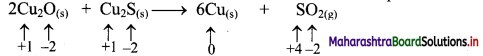b. Identify the species that undergoes change in oxidation number.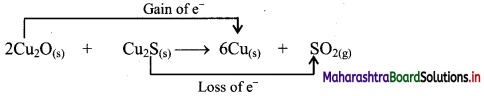c. The oxidation number of S increases from -2 to +4 and that of Cu decreases from +1 to 0. Because oxidation number of one species increases and that of the other decreases, the reaction is a redox reaction.
d. The oxidation number of S increases by loss of electrons and therefore, S is a reducing agent and it itself is oxidised. On the other hand, the oxidation number of Cu decreases by gain of electrons and therefore, Cu is an oxidising agent and itself is reduced.

Result:

1. The given reaction is a redox reaction.
2. Oxidant/oxidising agents (Reduced species): Cu2O/ Cu2S
3. Reductant/reducing agent (Oxidised species): Cu2S

[Note: Cu in both Cu2O and Cu2S undergoes reduction. Hence, both Cu2O and Cu2S can be termed as oxidising agents in the given reaction.]

ii. HF(aq) + OH(aq) → H2O(l) + F(aq)
a. Write oxidation number of all the atoms of reactants and products.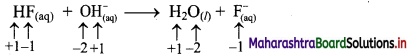b. Since, the oxidation numbers of all the species remain same, this is NOT a redox reaction. Result:
The given reaction is NOT a redox reaction.

iii. I2(aq) + 2 S2O32-(aq) → S4O62-(aq) + 2I(aq)
a. Write oxidation number of all the atoms of reactants and products.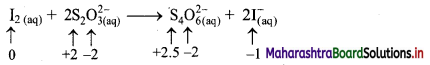b. Identify the species that undergoes change in oxidation number.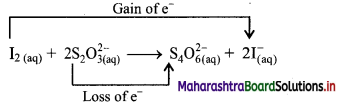c. The oxidation number of S increases from +2 to +2.5 and that of I decreases from 0 to -1. Because oxidation number of one species increases and that of the other decreases, the reaction is a redox reaction.
d. The oxidation number of S increases by loss of electrons and therefore, S is a reducing agent and itself is oxidised. On the other hand, the oxidation number of I decreases by gain of electrons and therefore, I is an oxidising agent and itself is reduced.

Result:

1. The given reaction is a redox reaction.
2. Oxidant/oxidising agent (Reduced species): I2
3. Reductant/reducing agent (Oxidised species): S2O32-

Question E.
What is oxidation? Which one of the following pairs of species is in its oxidized state ?
a. Mg / Mg2+
b. Cu / Cu2+
c. O2 / O2-
d. Cl2 / Cl
a. Mg / Mg2+
Here, Mg loses two electrons to form Mg2+ ion.
$$\mathrm{Mg}_{(\mathrm{s})} \longrightarrow \mathrm{Mg}_{(\mathrm{aq})}^{2+}+2 \mathrm{e}^{-}$$
Hence, Mg / Mg2+ is an oxidized state.

b. Cu/Cu2+
Here, Cu loses two electrons to form Cu2+ ion.
$$\mathrm{Cu}_{(\mathrm{s})} \longrightarrow \mathrm{Cu}_{(\mathrm{aq})}^{2+}+2 \mathrm{e}^{-}$$
Hence, Cu/Cu2+ is in an oxidized state.

c. O2 / O2-
Here, each O gains two electrons to form O2- ion.
$$\mathrm{O}_{2(\mathrm{~g})}+4 \mathrm{e}^{-} \longrightarrow 2 \mathrm{O}_{(\mathrm{aq})}^{2-}$$
Hence, O2 / O2- is in a reduced state.

d. Cl2 / Cl
Here, each Cl gains one electron to form Cl ion.
$$\mathrm{Cl}_{2(\mathrm{~g})}+2 \mathrm{e}^{-} \longrightarrow 2 \mathrm{Cl}_{(\mathrm{aq})}^{-}$$
Hence, Cl2 / Cl is in a reduced state.Question F.
Justify the following reaction as redox reaction.
2 Na2(s) + S(s) → Na2S(s)
Find out the oxidizing and reducing agents.
i. Redox reaction can be described as electron transfer as shown below:
2Na(s) + S(s) → 2Na+ + S2-
ii. Charge development suggests that each sodium atom loses one electron to form Na+ and sulphur atom gains two electrons to form S2-. This can be represented as follows: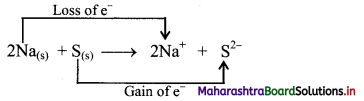iii. When Na is oxidised to Na2S, the neutral Na atom loses electrons to form Na+ in Na2S while the elemental sulphur gains electrons and forms S2- in Na2S.
iv. Each of the above steps represents a half reaction which involves electron transfer (loss or gain).
v. Sum of these two half reactions or the overall reaction is a redox reaction.
vi. Oxidising agent is an electron acceptor and hence, S is an oxidising agent. Reducing agent is an electron donor and hence, Na is a reducing agent.

Question G.
Provide the stock notation for the following compounds : HAuCl4, Tl2O, FeO, Fe2O3, MnO and CuO.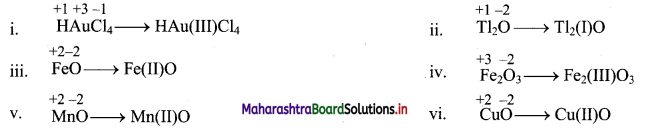Question H.
Assign oxidation number to each atom in the following species.
a. Cr(OH)4
b. Na2S2O3
c. H3BO3
i. Cr(OH)4
Oxidation number of O = -2
Oxidation number of H = +1
Cr(OH)4 is an ionic species.
∴ Sum of the oxidation numbers of all atoms = – 1
∴ Oxidation number of Cr + 4 × (Oxidation number of O) + 4 × (Oxidation number of H) = – 1
∴ Oxidation number of Cr + 4 × (-2) + 4 × (+1) = – 1
∴ Oxidation number of Cr – 8 + 4 = – 1
∴ Oxidation number of Cr – 4 = – 1 –
∴ Oxidation number of Cr = – 1 + 4
∴ Oxidation number of Cr in Cr(OH)4 = +3

ii. Na2S2O3
Oxidation number of Na = +1
Oxidation number of O = -2
Na2S2O3 is a neutral molecule.
∴ Sum of the oxidation numbers of all atoms = 0
∴ 2 × (Oxidation number of Na) + 2 × (Oxidation number of S) + 3 × (Oxidation number of O) = 0
∴ 2 × (+1) + 2 × (Oxidation number of S) + 3 × (-2) = 0
∴ 2 × (Oxidation number of S) + 2 – 6 = 0
∴ 2 × (Oxidation number of S) = + 4
∴ Oxidation number of S = +$$\frac {4}{2}$$
∴ Oxidation number of S in Na2S2O3 = +2

iii. H3BO3
Oxidation number of H = +1
Oxidation number of O = -2
H3BO3 is a neutral molecule.
∴ Sum of the oxidation numbers of all atoms = 0
∴ 3 × (Oxidation number of H) + (Oxidation number of B) + 3 × (Oxidation number of O) = 0
∴ 3 × (+1) + (Oxidation number of B) + 3 × (-2) = 0
∴ Oxidation number of B + 3 – 6 = 0
∴ Oxidation number of B in H3BO3 = +3

Question I.
Which of the following redox couple is stronger oxidizing agent ?
a. Cl2 (E0 = 1.36 V) and Br2 (E0 = 1.09 V)
b. $$\mathrm{MnO}_{4}^{\Theta}$$ (E0 = 1.51 V) and $$\mathrm{Cr}_{2} \mathrm{O}_{7}^{2 \Theta}$$ (E0 = 1.33 V)
a. Cl2 has a larger positive value of E0 than Br2. Thus, Cl2 is a stronger oxidizing agent than Br2.
b. $$\mathrm{MnO}_{4}^{\Theta}$$ has larger positive value of E0 than $$\mathrm{Cr}_{2} \mathrm{O}_{7}^{2 \Theta}$$. Thus, $$\mathrm{MnO}_{4}^{\Theta}$$ is stronger oxidizing agent than $$\mathrm{Cr}_{2} \mathrm{O}_{7}^{2 \Theta}$$

Question J.
Which of the following redox couple is stronger reducing agent ?
a. Li (E0 = – 3.05 V) and Mg(E0 = – 2.36 V)
b. Zn(E0 = – 0.76 V) and Fe(E0 = – 0.44 V)
a. Li has a larger negative value of E0 than Mg. Thus, Li is a stronger reducing agent than Mg.
b. Zn has a larger negative value of E0 than Fe. Thus, Zn is a stronger reducing agent than Fe.4. Balance the reactions/equations :

Question A.
Balance the following reactions by oxidation number method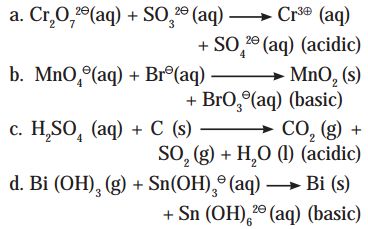i. $$\mathrm{Cr}_{2} \mathrm{O}_{7(\mathrm{aq})}^{2-}+\mathrm{SO}_{3(\mathrm{aq})}^{2-} \longrightarrow \mathrm{Cr}_{(\mathrm{aq})}^{3+}+\mathrm{SO}_{4(\mathrm{aq})}^{2-} \quad(\text { acidic })$$
Step 1: Write skeletal equation and balance the elements other than O and H.
$$\mathrm{Cr}_{2} \mathrm{O}_{7(a q)}^{2-}+\mathrm{SO}_{3(a)}^{2-} \longrightarrow 2 \mathrm{Cr}_{(\mathrm{aq})}^{3+}+\mathrm{SO}_{4(\mathrm{aq})}^{2-}$$
Step 2: Assign oxidation number to Cr and S. Calculate the increase and decrease in the oxidation number and make them equal.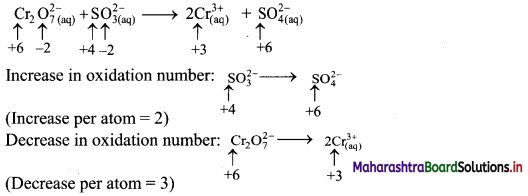To make the net increase and decrease equal, we must take 3 atoms of S and 2 atoms of Cr. (There are already 2 Cr atoms.)
Step 3: Balance ‘O’ atoms by adding 4H2O to the right-hand side.
$$\mathrm{Cr}_{2} \mathrm{O}_{7(\mathrm{aq})}^{2-}+3 \mathrm{SO}_{3(\mathrm{aq})}^{2-} \longrightarrow 2 \mathrm{Cr}_{(\mathrm{aq})}^{3+}+3 \mathrm{SO}_{4(\mathrm{aq})}^{2-}+4 \mathrm{H}_{2} \mathrm{O}_{(l)}$$
Step 4: The medium is acidic. To make the charges and hydrogen atoms on the two sides equal, add 8H on the left-hand side.
$$\mathrm{Cr}_{2} \mathrm{O}_{7(\mathrm{aq})}^{2-}+3 \mathrm{SO}_{3(\mathrm{aq})}^{2-}+8 \mathrm{H}_{(\mathrm{aq})}^{+} \longrightarrow 2 \mathrm{Cr}_{(\mathrm{aq})}^{3+}+3 \mathrm{SO}_{4(\mathrm{aq})}^{2-}+4 \mathrm{H}_{2} \mathrm{O}_{(l)}$$
Step 5: Check two sides for balance of atoms and charges.
Hence, balanced equation: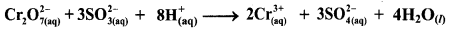ii. $$\mathrm{MnO}_{4(\mathrm{aq})}^{-}+\mathrm{Br}_{(\mathrm{aq})}^{-} \longrightarrow \mathrm{MnO}_{2(\mathrm{~s})}+\mathrm{BrO}_{3}^{-}{(a q)} \quad \text { (basic) }$$
Step 1: Write skeletal equation and balance the elements other than O and H.
$$\mathrm{MnO}_{4(\mathrm{aq})}^{-}+\mathrm{Br}_{(\mathrm{aq})}^{-} \longrightarrow \mathrm{MnO}_{2(\mathrm{~s})}+\mathrm{BrO}_{3}^{-}{ }_{(\mathrm{aq})}$$
Step 2: Assign oxidation number to Mn and Br. Calculate the increase and decrease in the oxidation number and make them equal.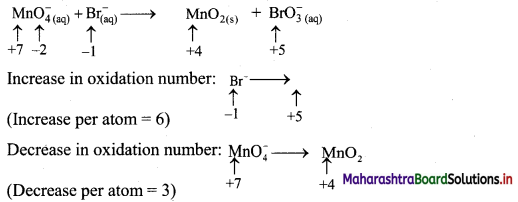To make the net increase and decrease equal, we must take 2 atoms of Mn.
$$2 \mathrm{MnO}_{4(\mathrm{aq})}^{-}+\mathrm{Br}_{(\mathrm{aq})}^{-} \longrightarrow 2 \mathrm{MnO}_{2(\mathrm{~s})}+\mathrm{BrO}_{3(\mathrm{aq})}^{-}$$
Step 3: Balance ‘O’ atoms by adding H2O to the right-hand side.
$$2 \mathrm{MnO}_{4(a q)}^{-}+\mathrm{Br}_{(2 q)}^{-} \longrightarrow 2 \mathrm{MnO}_{2(\mathrm{~s})}+\mathrm{BrO}_{3 \text { (aq) }}^{-}+\mathrm{H}_{2} \mathrm{O}_{(l)}$$
Step 4: The medium is basic. To make the charges and hydrogen atoms on the two sides equal, add 2H+ on the left-hand side.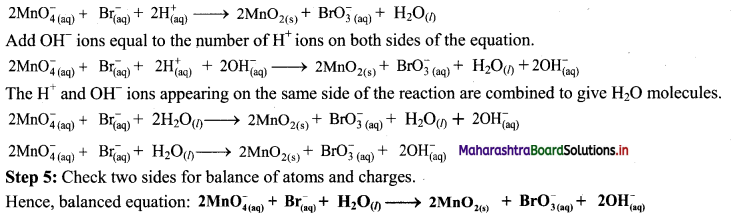iii. H2SO4(aq) + C(s) → CO2(g) + SO2(g) + H2O(l) (acidic)
Step 1: Write skeletal equation and balance the elements other than O and H.
H2SO4(aq) + C(s) → CO2(g) + SO2(g) + H2O(l)
Step 2: Assign oxidation number to S and C. Calculate the increase and decrease in the oxidation number and make them equal.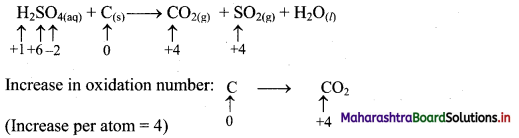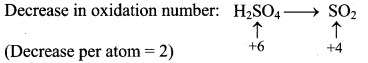To make the net increase and decrease equal, we must take 2 atoms of S.
2H2SO4(aq) + C(s) → CO2(g) + 2SO2(g) + H2O(l)
Step 3: Balance ‘O’ atoms by adding H2O to the right-hand side.
2H2SO4(aq) + C(s) → CO2(g) + 2SO2(g) + H2O(l) + H2O(l)
Step 4: The medium is acidic. There is no charge on either side. Hydrogen atoms are equal on both side.
2H2SO4(aq) + C(s) → CO2 + 2SO2(g) + H2O(l)
Step 5: Check two sides for balance of atoms and charges.
Hence, balanced equation: 2H2SO4(aq) + C(s) → CO2(g) + 2SO2(g) + H2O(l)

iv. $$\mathrm{Bi}(\mathrm{OH})_{3(\mathrm{~s})}+\mathrm{Sn}(\mathrm{OH})_{3(\mathrm{aq})}^{-} \longrightarrow \mathrm{Bi}_{(\mathrm{s})}+\mathrm{Sn}(\mathrm{OH})_{6(\mathrm{aq})}^{2-}$$ (basic)
Step 1: Write skeletal equation and balance the elements other than O and H.
$$\mathrm{Bi}(\mathrm{OH})_{3(\mathrm{~s})}+\mathrm{Sn}(\mathrm{OH})_{3(\mathrm{aq})}^{-} \longrightarrow \mathrm{Bi}_{(\mathrm{s})}+\mathrm{Sn}(\mathrm{OH})_{6(\mathrm{aq})}^{2-}$$
Step 2: Assign oxidation numbers to Bi and Sn. Calculate the increase and decrease in the oxidation number and make them equal.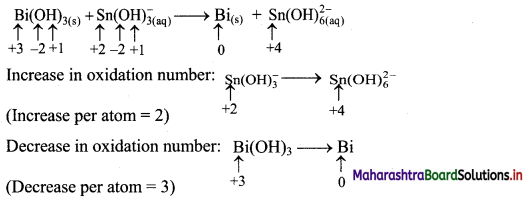To make the net increase and decrease equal, we must take 3 atoms of Sn and 2 atoms of Bi.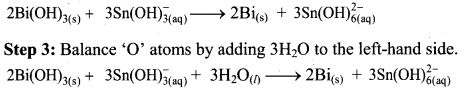Step 4: The medium is basic. To make hydrogen atoms on the two sides equal, add 3W on the right-hand side.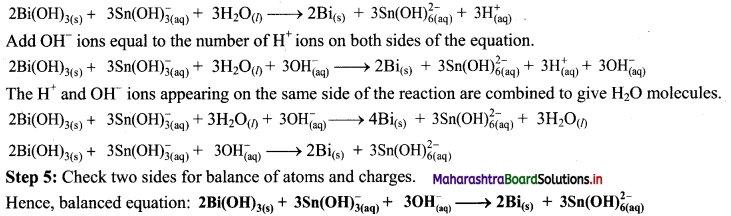Question B.
Balance the following redox equation by half reaction method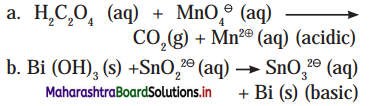i. H2C2O4(aq) + $$\mathrm{MnO}_{4(a q)}^{-}$$ → CO2(g) + $$\mathrm{Mn}_{(\mathrm{aq})}^{2+}$$
Step 1: Write unbalanced equation for the redox reaction. Assign oxidation number to all the atoms in reactants and products. Divide the equation into two half equations.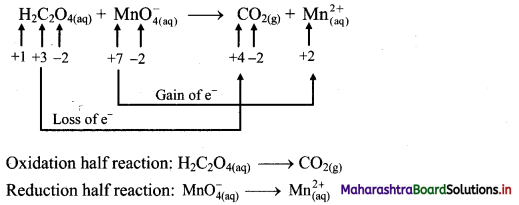Step 2: Balance the atoms except O and H in each half equation. Balance half equation for O atoms by adding 4H2O to the right side of reduction half equation.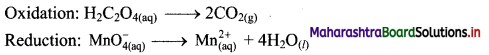Step 3: Balance H atoms by adding H+ ions to the side with less H. Hence, add 2H+ ions to the right side of oxidation half equation and 8H+ ions to the left side of reduction half equation.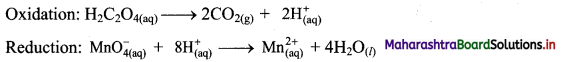Step 4: Now add 2 electrons to the right side of oxidation half equation and 5 electrons to the left side of reduction half equation to balance the charges.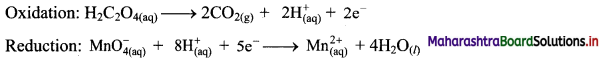Step 5: Multiply oxidation half equation by 5 and reduction half equation by 2 to equalize number of electrons in two half equations. Then add two half equation.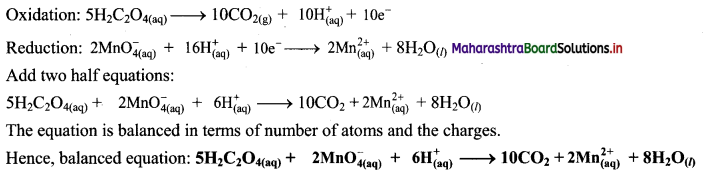ii. $$\mathrm{Bi}(\mathrm{OH})_{3(\mathrm{~s})}+\mathrm{SnO}_{2(\mathrm{aq})}^{2-} \longrightarrow \mathrm{SnO}_{3(\mathrm{aq})}^{2-}+\mathrm{Bi}_{(\mathrm{s})}$$
Step 1: Write unbalanced equation for the redox reaction. Assign oxidation number to all the atoms in reactants and products. Divide the equation into two half equations.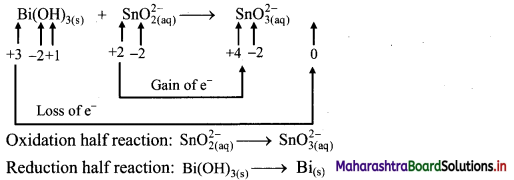Step 2: Balance half equations for O atoms by adding H2O to the side with less O atoms. Add 1H2O to left side of oxidation half equation and 3H2O to the right side of reduction half equation.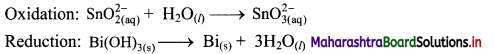Step 3: Balance H atoms by adding H+ ions to the side with less H. Hence, add 2H+ ions to the right side of oxidation half equation and 3H+ ions to the left side of reduction half equation.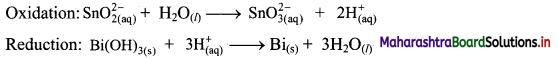Step 4: Now add 2 electrons to the right side of oxidation half equation and 3 electrons to the left side of reduction half equation to balance the charges.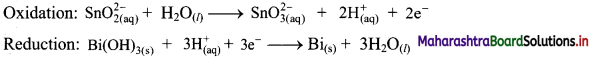Step 5: Multiply oxidation half equation by 3 reduction half equation by 2 to equalize number of electrons in two half equations. Then add two half equation.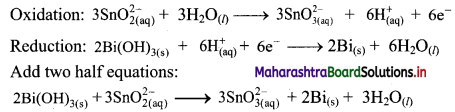Reaction occurs in basic medium. However, H+ ions cancel out and the reaction is balanced. Hence, no need to add OH ions. The equation is balanced in terms of number of atoms and the charges.
Hence, balanced equation: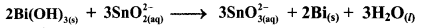5. Complete the following table :

Assign oxidation number to the underlined species and write Stock notation of compound

 Compound Oxidation number Stock notation AuCl3 …………….. …………….. SnCl2 …………….. …………….. $$\underline{\mathrm{V}}_{2} \mathrm{O}_{7}^{4-}$$ …………….. …………….. $$\underline{\mathrm{Pt}} \mathrm{Cl}_{6}^{2-}$$ …………….. …………….. H3AsO3 …………….. ……………..

 Compound Oxidation number Stock notation AuCl3 +3 Au(III)Cl3 SnCl2 +2 Sn(II)Cl2 $$\underline{\mathrm{V}}_{2} \mathrm{O}_{7}^{4-}$$ +5 V2(V)$$\mathrm{O}_{7}^{4-}$$ $$\underline{\mathrm{Pt}} \mathrm{Cl}_{6}^{2-}$$ +4 Pt(IV)$$\mathrm{Cl}_{6}^{2-}$$ H3AsO3 +3 H3As(III)O3

11th Chemistry Digest Chapter 6 Redox Reactions Intext Questions and Answers

Can you tell? (Textbook Page No. 81)

Question i.
Why does cut apple turn brown when exposed to air?
Cut apple turns brown when exposed to air because polyphenols are released. These polyphenols undergo oxidation in the presence of air and impart brown colour.

Question ii.
Why does old car bumper change colour?
Car bumper is made of iron which undergoes rusting over a period of time. Hence, old car bumper changes colour.

Question iii.
Why do new batteries become useless after some days?
Batteries generate electricity by redox reactions. Once the chemicals taking part in redox reaction are used up, the battery cannot generate power. Hence, new batteries become useless after some days.Can you recall? (Textbook Page No. 81)

Question i.
What is combustion reaction?
Combustion is a process in which a substance combines with oxygen.

Question ii.
Write an equation for combustion of methane.
Combustion of methane: CH4 + 2O2 → CO2 + 2H2O + Heat + Light

Question iii.
What is the driving force behind reactions of elements?
The ability of element to combine with other element or the ability of element to replace other element in compound is the driving force behind the reactions. This may involve formation of precipitates, formation of water, release of gas, etc.

Try this. (Textbook Page No. 82)

Question 1.
Complete the following table of displacement reactions. Identify oxidising and reducing agents involved.

 Reactants Products Zn(s) + ————(aq) ————-(aq) + Cu(s) Cu(s) + 2Ag+(aq) —————– + ————– ———– + ————- $$\mathrm{Co}_{(\mathrm{aq})}^{2+}$$ + Ni(s)